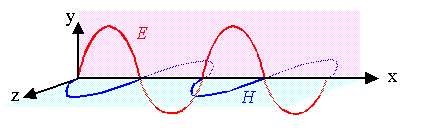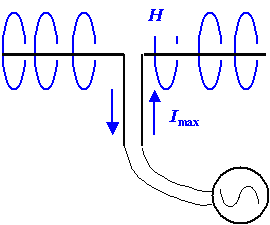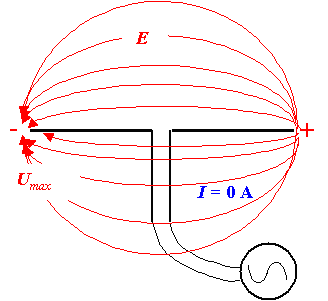Here is the relevant picture and the questions going with it from subchapter 5.1.4:Ey(t)  = Ey0 · exp{i(kxwt)}
Ez(t)  = Hz0 · exp{i(kxwt)}
1. Is the electrical and magnetic field really in phase as shown above?
2. Can polarization be described by a vector? Could I just use a unit vector in E0-direction?
3. How do I describe polarization in the particle picture?
4. How large is the electrical field E0 in a typical light wave. How large (roughly) is it for one photon of given energy?
5. Let's assume you know the electrical field E0: how large is the magnetic field H0 going with it?Let's consider some answers1. Is the electrical and magnetic field really in phase as shown in the drawing above?Yes it is - all serious sources agree even so the reasons given are mostly not obvious. The real question, of course, is: why should this be a question at all?Solving the Maxwell equations quite generally for the "wave" case, simply gives solutions with no phase differences of the form:
E(r,t); H(r,t)   = E0; H0 · exp{i(krwt)}True enough. But this simple solution describes an infinitely extended electromagnetic plane wave—something that does not exist. So let's look what happens for finite waves that have a beginning at some point in space.Not so easy for light. The generation of a photon is always a quantum mechanical effect not covered by the Maxwell equations. However, the equation above is valid for all electromagnetic waves. It is also valid, e.g. for the radio waves produced by a simple dipole antenna and this is covered by the Maxwell equations. We thus can look at the generation of a radio wave instead of a light wave; something easier to conceive than the generation of a light wave by the transition of an electron from one energy level to another one.The figure below shows the principle. An AC current is fed to a dipole, it drives charges to the two ends. Maximum current flows when there are no charges at the dipole ends, we then have maximum magnetic fields and no electric fieldThe charges transported to the end of the dipole cause an electrical field that opposes current flow. Eventually, at maximum charge and thus electrical field, current flow is zero and there is no magnetic field.The unavoidable conclusion is that at the place where we make the wave - and it doesn't matter what kind of wavelength you envision - the electrical field and the magnetic field are 90o out of phase!Now we have a problem: far away from the "light source" the two fields are in phase but where we make them they are out of phase!The problem is solved when one looks at the full set of equations (not easy). Far away from the dipole, in the far field, we "see" a point source that emits a spherical wave and there is no phase difference, indeed. Close to the dipole (measured in units of the wavelength), in the near field, we do not see a point source but a complex geometry as sketched above. The fields in the near field do not have spherical symmetry and phases right at the dipole are different by 90o, indeed.In mathematical terms, we superimpose a complicated near field term with a general 1/rn dependence (n > 1 on a simple spherical wave. The near-field term dominates close to the antenna. Since it decreases faster than the spherical wave term, for large distances we can neglect the near-field term and are left with a spherical wave that appears to be a plane wave if you only look in parts of space.You don't need to know this but you should be aware that as soon as you leave the ideal realm of the simple plane wave without a beginning and an end, things tend to get much more complicated. But things also tend to be much more like what we actually know.2. Can polarization be described by a vector? Could I just use a unit vector in E0-direction?Yes, you can describe polarization by vector. But beware! Polarization in general is more tricky than you might think and a simple vector is not always enough to describe it mathematically. (Remember the problems encountered in using a polar vector for describing rotations?). You will need a tensor / matrix if you go about it systematically.That was more or less a quote from here.3. How do I describe polarization in the particle picture?You don't. You look at the spin of the particle instead.Read more about it here.4. How large is the electrical field E0 in a typical light wave. How large (roughly) is it for one photon of given energy?The first question can be answered without too much trouble; the second question is tricky.Look at the exercise to these (and other) questions. Try to do it. Then look a the solution.5. Let's assume you know the electrical field E0: how large is the magnetic field H0 going with it?Here is the relevant equation.5.1.4 Energy Flow, Poynting Vector and PolarizationExercise 5.1.2 Energy, Field strength and PhotonsSolution to Exercise 5.1-2: Energy, Field strength and Photons